# Predict the total CO2 emissions a car produces using machine learning

Nowadays, it is essential to reduce CO2 emissions in the atmosphere to fight against climate change. Indeed, many laws forbid cars whose CO2 emissions are above a specific limit; they must be below it to be approved to drive through the roads.

That is why this machine learning example is a topical issue that should raise awareness in the reader.

Performance optimization can be applied to understand the behavior of car engines and their CO2 emissions.

For this study, we have gathered an extensive data set of different types of cars that could exist in any place in the world.

We will predict CO2 emissions with certain independent variables. Any car company could adjust this value by simply modifying different parameters like the size of the engine and many other engines features.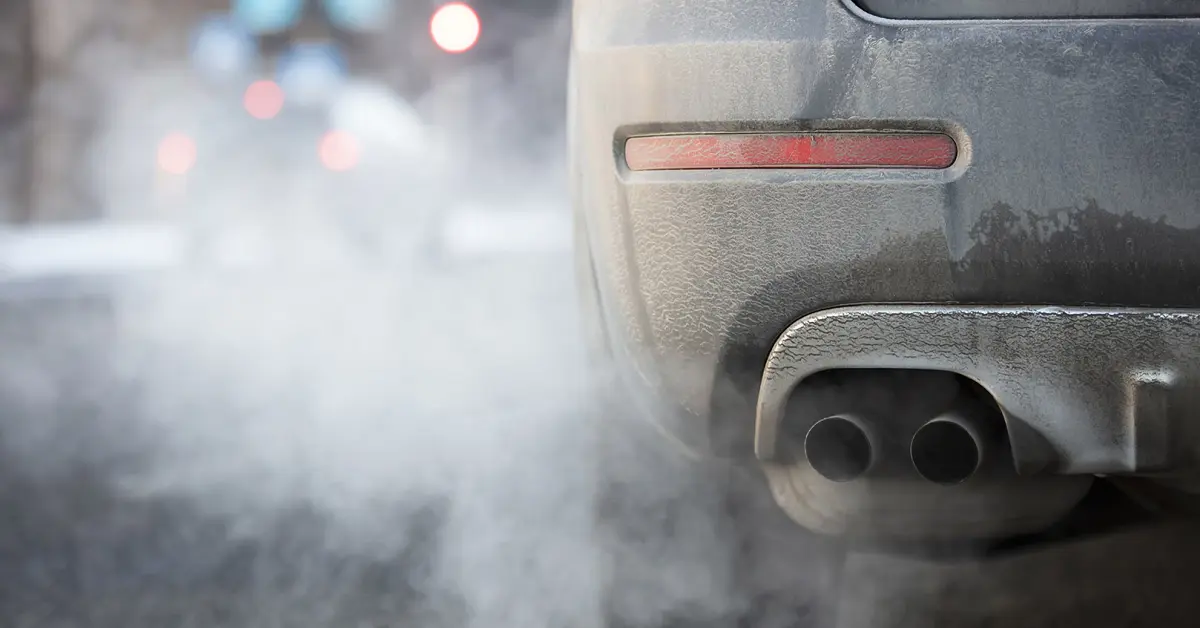### Contents:

1. Application type.
2. Data set.
3. Neural network.
4. Training strategy.
5. Model selection.
6. Testing analysis.
7. Model deployment.

This example is solved with Neural Designer. To follow it step by step, you can use the free trial.

## 1. Application type

This is an approximation project since the variable to be predicted is continuous (CO2 emissions).

The fundamental goal here is to model the CO2 emissions as a function of several car engines features.

## 2. Data set

The first step is to prepare the data set, which is the source of information for the approximation problem. It is composed of:

• Data source.
• Variables.
• Instances.

The file co2_emissions.csv contains the data for this example. Here the number of variables (columns) is 12, and the number of instances (rows) is 7385.

In that way, this problem has the 12 following variables:

• make, car brand under study.
• model, the specific model of the car.
• vehicle_class, car body type of the car.
• engine_size, size of the car engine, in Litres.
• cylinders, number of cylinders.
• transmission, "A" for 'Automatic', "AM" for 'Automated manual', "AS" for 'Automatic with select shift', "AV" for 'Continuously variable', "M" for 'Manual'.
• fuel_type, "X" for 'Regular gasoline', "Z" for 'Premium gasoline', "D" for 'Diesel', "E" for 'Ethanol (E85)', "N" for 'Natural gas'.
• fuel_consumption_city, City fuel consumption ratings, in litres per 100 kilometres.
• fuel_consumption_hwy, Highway fuel consumption ratings, in litres per 100 kilometres.
• fuel_consumption_comb(l/100km), the combined fuel consumption rating (55% city, 45% highway), in L/100 km.
• fuel_consumption_comb(mpg), the combined fuel consumption rating (55% city, 45% highway), in miles per gallon (mpg).
• co2_emissions, the tailpipe emissions of carbon dioxide for combined city and highway driving, in grams per kilometre.

All the variables that appear in the study are inputs, except for 'make' and 'model' that have to stay as "unused", and 'co2_emissions', which is the output that we want to extract from this machine learning study.

Also, we realized that the instance 'vehicle_class' could be a bit problematic to analyze because it contains many categorical variables inside. Still, in a further study, we can see that the final selection error is higher without this variable than including it. Therefore, as one of the main goals of a Neural Network study is to minimize the Selection Error as much as possible, we can add 'vehicle_class' as an input.

As we can see, there are certain variables that should stay as "unused" too. That is the case of 'fuel_consumption' (in city, highway, or combined). These instances could be targets of the study (CO2 and fuel consumption are dependent on each other), but it is more visual to see the CO2 emissions as the final output.

They are divided at random into training, selection, and testing subsets, containing 60%, 20%, and 20% of the instances, respectively. More specifically, 4431 samples are used here for training, 1477 for validation, and 1477 for testing.

Once all the data set information has been set, we will perform some analytics to check the quality of the data.

For instance, we can calculate the data distribution. The next figure depicts the histogram for the target variable.As we can see in the diagram, the CO2 emissions have a normal distribution. In fact, there are a lot of cars that have median CO2 emissions that stay below the limit we were talking about before. But, on the other hand, there is exactly 0.108 percent of the total cars in this study that have very high CO2 emissions that nowadays would not be able to drive.

The next figure depicts inputs-targets correlations. This might help us to see the influence of the different inputs on the final car emissions.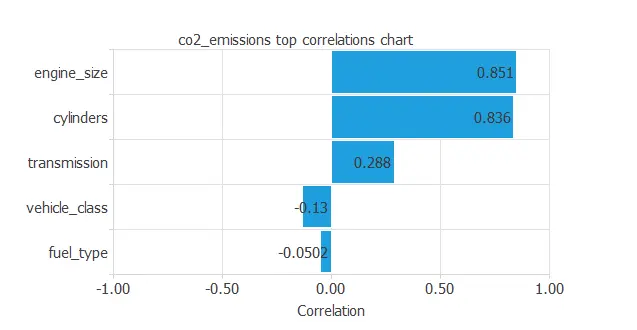The above chart shows that a few instances have an important dependency on CO2 emissions. As we can see, "engine_size" and "cylinders" depend positively on the CO2 emissions; that means the bigger the engine size, the more CO2 the car emits, for example. On the other hand, some instances have a negative dependency on the emissions.

We can also plot a scatter chart with the CO2 emissions versus the engine size.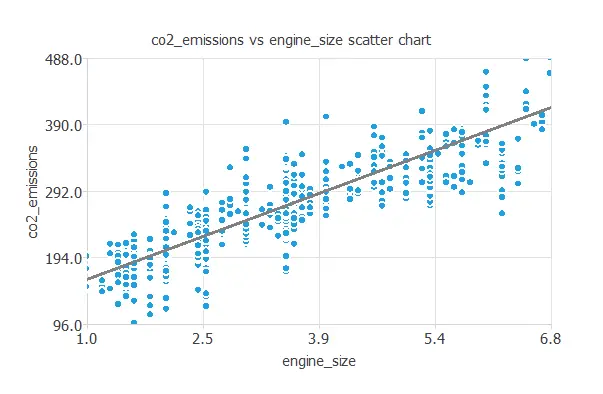In general, the more engine size, the more emissions. However, CO2 emissions depend on all the inputs at the same time.

## 3. Neural network

The neural network will output the CO2 emissions as a function of consumption, transmission and other car engine features.

For this approximation example, the neural network is composed of:

• Scaling layer.
• Perceptron layers.
• Unscaling layer.

The scaling layer transforms the original inputs to normalized values. Here the mean and standard deviation scaling method is set so that the input values have a mean of 0 and a standard deviation of 1.

Here two perceptron layers are added to the neural network. This number of layers is enough for most applications. The first layer has 28 inputs and 3 neurons. The second layer has 3 inputs and 1 neuron.

The unscaling layer transforms the normalized values from the neural network into the original outputs. Here the mean and standard deviation unscaling method will also be used.

The next figure shows the resulting network architecture.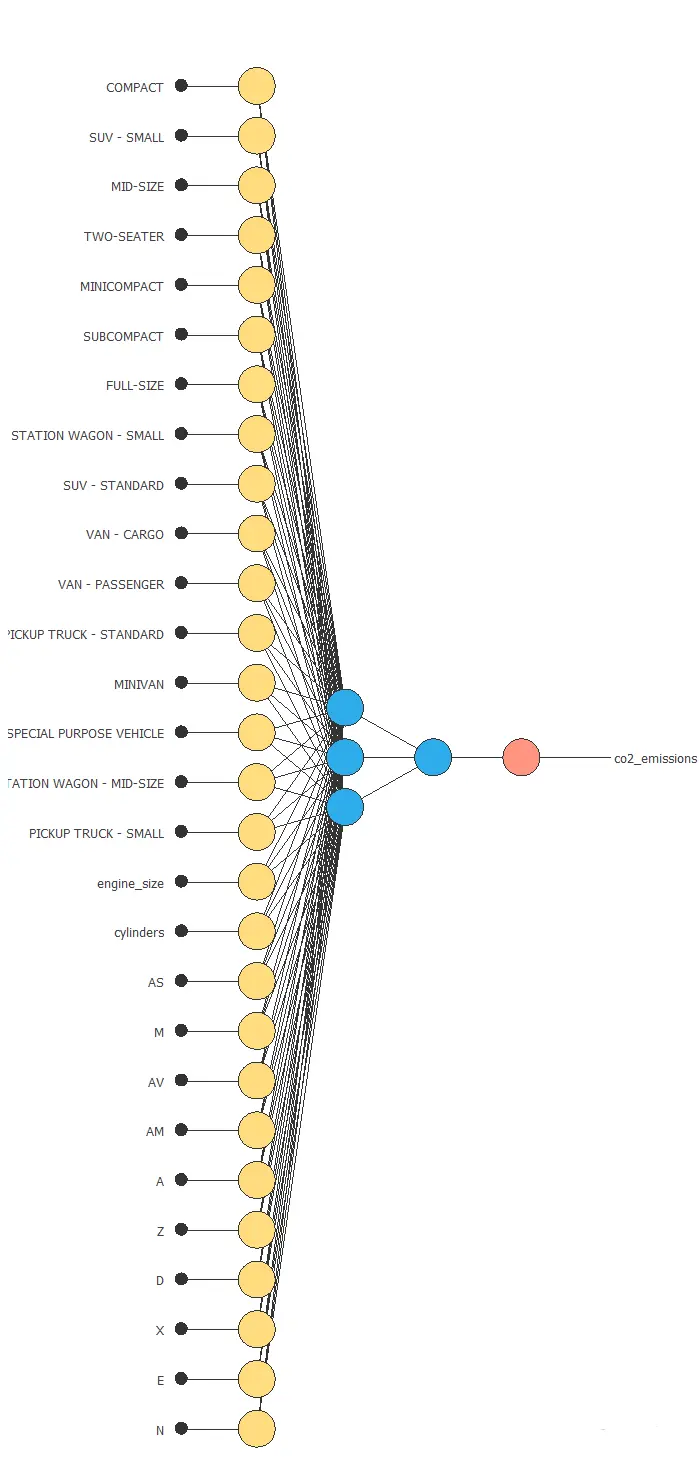## 4. Training strategy

The next step is to select an appropriate training strategy, which defines what the neural network will learn. A general training strategy is composed of two concepts:

• A loss index.
• An optimization algorithm.

The loss index chosen is the normalized squared error with L2 regularization. This loss index is the default in approximation applications.

The optimization algorithm chosen is the quasi-Newton method. This optimization algorithm is the default for medium-sized applications like this one.

Once the strategy has been set, we can train the neural network. The following chart shows how the training (blue) and selection (orange) errors decrease with the training epoch during the training process.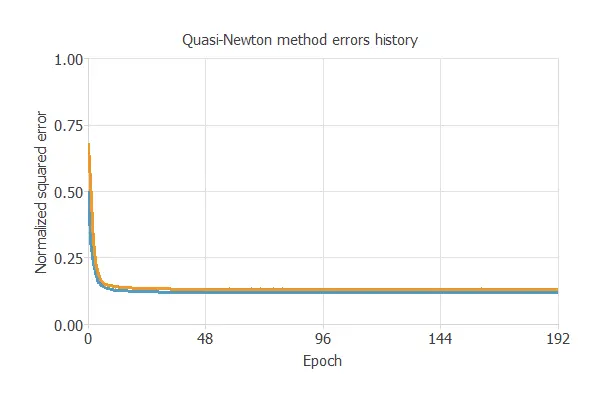The most important training result is the final selection error. Indeed, this is a measure of the generalization capabilities of the neural network. Here the final selection error is selection error = 0.145 NSE.

## 5. Model selection

The objective of model selection is to find the network architecture with the best generalization properties. That is, we want to improve the final selection error obtained before (0.145 NSE).

The best selection error is achieved by using a model whose complexity is the most appropriate to produce an adequate data fit. Order selection algorithms are responsible for find the optimal number of perceptrons in the neural network.

The final selection error takes a minimum value at some point. Here, the optimal number of neurons is 10, corresponding to a selection error of 0.131.

## 6. Testing analysis

The objective of the testing analysis is to validate the generalization performance of the trained neural network. The testing compares the values provided by this technique to the observed values.

A standard testing technique in approximation problems is to perform a linear regression analysis between the predicted and the real values, using an independent testing set. The next figure illustrates a graphical output provided by this testing analysis.From the above chart, we can see that the neural network is predicting well the entire range of the CO2 emissions data. The correlation value is R2 = 0.924, which is very close to 1.

## 7. Model deployment

The model is now ready to estimate the CO2 emissions of a certain car with satisfactory quality over the same data range.

We can plot a directional output of the neural network to see how the emissions vary with a given input for all other inputs fixed. The next plot shows the CO2 emissions as a function of the number of cylinders, through the following point:

• vehicle_class: COMPACT.
• engine_size: 3.16 L.
• cylinders: 5.
• transmission: AS.
• fuel_type: Z.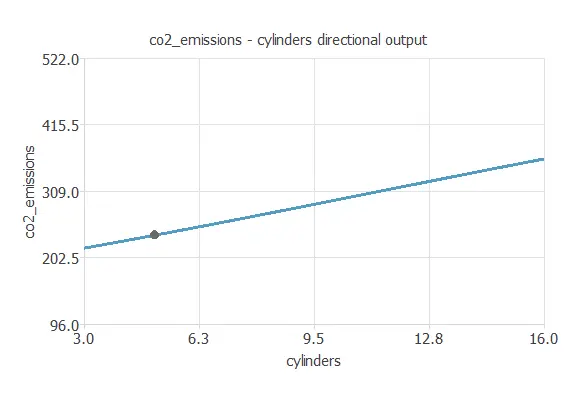The co2-emissions.py contains the Python code for the CO2 emissions.